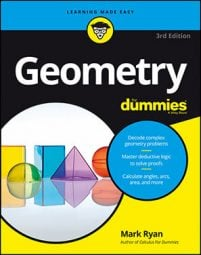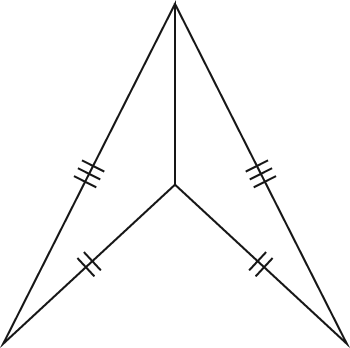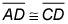##### Geometry For DummiesAlthough it sounds like a top-secret government department, CPCTC is actually just an acronym for a statement about congruent triangles: Corresponding Parts of Congruent Triangles are Congruent.

As you'll see in the following example, CPCTC is very useful when working with proofs. But first, you'll need the following property to do the problem. (It's an incredibly simple concept that comes up in many proofs.)

The Reflexive Property: The Reflexive Property states that any segment or angle is congruent to itself. (Who would've thought?)

Whenever you see two triangles that share a side or an angle, that side or angle belongs to both triangles. With the Reflexive Property, the shared side or angle becomes a pair of congruent sides or angles that you can use as one of the three pairs of congruent things that you need to prove the triangles congruent. Okay, now onto the example.Using the Reflexive Property for the shared side, these triangles are congruent by SSS.

And here's the proof diagram.

Before you write out the formal proof, you need to come up with a game plan. Here's one possibility:

• Look for congruent triangles. The congruent triangles should just about jump out at you from this diagram. Think about how you'll show that they're congruent. The triangles share side BD, giving you one pair of congruent sides. Side BD is an altitude, so that gives you congruent right angles. And because side BD is a median,That does it; you have SAS.

• Now think about what you have to prove and what you'd need to know to get there.

And how will you get that? Why, with CPCTC, of course!

Here's the two-column proof:

Every little step in a proof must be spelled out. For instance, in this proof, you can't go from the idea of a median (line 1) to congruent segments (line 3) in one step—even though it's obvious—because the definition of median says nothing about congruent segments. By the same token, you can't go from the idea of an altitude (line 4) to congruent right angles (line 7) in one step or even two steps. You need three steps to connect the links in this chain of logic: